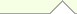# Free Discrete Mathematics Books

 Abstract AlgebraLinear AlgebraCommutative AlgebraComplex AlgebraElliptic CurvesGeometric AlgebraGroups TheoryHigher AlgebraHomological AlgebraLie AlgebraDifferential AlgebraRings and FiledsGeometryAlgebraic GeometryDifferential GeometryFractalsRiemannian GeometryMathematical AnalysisComplex AnalysisFunctional AnalysisDifferential AnalysisFourier AnalysisHarmonic AnalysisNumerical AnalysisReal AnalysisTopologyAlgebraic TopologyDifferential TopologyGeometric TopologyK-theoryApplied MathematicsCalculusDifferential EquationsCombinatoricsDiscrete MathematicsGraph TheoryNumber TheoryProbability TheorySet TheoryTrigonometryCategory TheoryConstants & Numerical SequencesBasic MathematicsClassical AnalysisHistory of MathematicsArithmetic GeometryMathematical SeriesModern GeometryBasic AlgebraDifferential EquationsHome About Us Link to us Sitemap Contact Us Post Queries### This section contains free e-books and guides on Discrete Mathematics, some of the resources in this section can be viewed online and some of them can be downloaded.

Discrete Mathematics BooksLecture Notes for College Discrete MathematicsGabor Horvath and Szabolcs TengelyPDF | 203 Pages | EnglishThis note explains the following topics: Sets, Sums and products, The Euclidean algorithm, Numeral systems, Counting, Proof techniques, Pascal's triangle, Recurrence sequences. Applied Discrete Mathematics William ShoaffWilliam D ShoaffOnline | NA Pages | EnglishThis note explains the following topics: positional and modular number systems, relations and their graphs, discrete functions, set theory, propositional and predicate logic, sequences, summations, mathematical induction and proofs by contradiction. Discrete Mathematics Lecture Notes Incomplete Preliminary VersionLaszlo BabaiPDF | 96 Pages | EnglishThis note covers the following topics: Logic, Asymptotic Notation, Convex Functions and Jensen�s Inequality, Basic Number Theory, Counting, Binomial coefficients, Graphs and Digraphs, Finite Probability Space, Finite Markov Chains. A Collection of Short Lectures on Discrete MathematicsWilliam D ShoaffOnline | NA Pages | EnglishThis note covers the following topics: Boolean Logic, Sets, Predicate Logic, Sequences, Recursion, Mathematical Induction, Relations, Functions, Naming Systems, Number Systems, Proofs. A Course in Discrete StructuresRafael Pass and Wei-Lung Dustin TsengPDF | 153 Pages | EnglishThis note covers the following topics: Sets, Functions and Relations, Proofs and Induction, Number Theory, Counting, Probability, Logic, Graphs, Finite Automata. Notes on Discrete Mathematics by James AspnesJames AspnesPDF | 390 Pages | EnglishThis is a course note on discrete mathematics as used in Computer Science. Topics covered includes: Mathematical logic, Set theory, The real numbers, Induction and recursion, Summation notation, Asymptotic notation, Number theory, Relations, Graphs, Counting, Linear algebra, Finite fields. Lectures In Discrete MathematicsEdward A. Bender and S. Gill WilliamsonOnline | NA Pages | EnglishThis note explains the following topics: Arithmetic, Logic and Numbers, Boolean Functions and Computer Arithmetic, Number Theory and Cryptography, Sets, Equivalence and Order, Functions, Induction, Sequences and Series, Lists, Decisions and Graphs, Basic Counting and Listing, Decision Trees, Basic Concepts in Graph Theory. Lecture Notes in Discrete MathematicsMarcel B. Finan, Arkansas Tech UniversityPDF | 224 Pages | EnglishThis note covers the following topics: fundamentals of mathematical logic , fundamentals of mathematical proofs , fundamentals of set theory , relations and functions , introduction to the Analysis of Algorithms, Fundamentals of Counting and Probability Theory and Elements of Graph Theory. Discrete Mathematics for Computer ScienceMike Clancy, David WagnerOnline | NA Pages | English The goal of this lecture note is to introduce students to ideas and techniques from discrete mathematics that are widely used in Computer Science. This note covers the following topics: Propositional logic, Induction, Strong induction, Structural induction, Proofs about algorithms, Algebraic algorithms, Number theory, RSA, Basics of counting, basic probability,Conditional probability, Linearity of expectation, variance. Discrete Mathematics (PDF 139P)L. Lovasz and K. VesztergombiPDF | 139 Pages | EnglishThis note covers the following topics: induction, counting subsets, Pascal's triangle, Fibonacci numbers, combinatorial probability, integers divisors and primes, Graphs, Trees, Finding the optimum, Matchings in graphs, Graph coloring. Discrete Mathematics with AlgorithmsM. O. Albertson and J. P. HutchinsonOnline | NA Pages | EnglishThis note covers the following topics: Sets and Algorithms, Arithmetic of Sets, Number Theory, Graph Theory, Searching and Sorting, Recurrence Relations. A Short Course in Discrete MathematicsEdward A. Bender and S. Gill WilliamsonOnline | NA Pages | EnglishThis book consists of six units of study: Boolean Functions and Computer Arithmetic, Logic, Number Theory and Cryptography, Sets and Functions, Equivalence and Order, Induction, Sequences and Series. Each of this is divided into two sections. Each section contains a representative selection of problems. These vary from basic to more difficult, including proofs for study by mathematics students or honors students. Discrete Maths IIHans CuypersPDF | 74 Pages | EnglishThis note explains the following topics: Relations, Maps, Order relations, Recursion and Induction, Bounding some recurrences, Graphs, Lattices and Boolean Algebras. Discrete Mathematics (with Kati Vesztergombi), 1999(PS) Introduction to Finite MathematicsJohn G. Kemeny, J. Laurie Snell, and Gerald L. ThompsonOnline | NA Pages | EnglishThis note covers the following topics: Compound Statements, Sets and subsets, Partitions and counting, Probability theory, Vectors and matrices, Linear programming and the theory of games, Applications to behavioral science problems. Discrete Mathematics Study Guide UVICDr. Gary MacGillivrayOnline | NA Pages | EnglishThis note covers the following topics: Logic and Foundations, Proposition logic and quantifiers, Set Theory, Mathematical Induction, Recursive Definitions, Properties of Integers, Cardinality of Sets, Pigeonhole Principle, Combinatorial Arguments, Recurrence Relations. Computational Discrete MathematicsK. SutnerOnline | NA Pages | EnglishThis note covers the following topics: Computation, Finite State Machines, Logic, SetsSet Theory, Three Theorems, Ordinals, Relations and Functions, Induction, Combinatorics, Algebra, Cellular Automata and FSRs. Fundamental Problems in Algorithmic AlgebraChee YapOnline | NA Pages | EnglishThis book explains the following topics: Arithmetic, The Greatest Common Divisor, Subresultants, Modular Techniques, Fundamental Theorem of Algebra, Roots of Polynomials, Sturm Theory, Gaussian Lattice Reduction, Lattice Reduction and Applications, Linear Systems, Elimination Theory, Groebner Bases, Bounds in Polynomial Ideal Theory and Continued Fractions. Introduction to Complexity ClassesChee K. YapOnline | NA Pages | EnglishThis book explains the following topics: Computability, Initiation to Complexity Theory, The Turing Model: Basic Results, Introduction to the Class NP, Reducibilities, Complete Languages, Separation Results, Stochastic Choices, Quantum Complexity, Theory of Real Computation and Kolmogorov Complexity. Exact Geometric Computation Basics of Algebra and Analysis for Computer ScienceSponsored linksHome  |  About Us  |  Sitemap  |  Contact Us  |  Link to us  |  Post Your Queries |© Copyright 2015-2016. FreeBookCentre.net, All Rights Reserved. Privacy Policy  |  Terms & Conditions